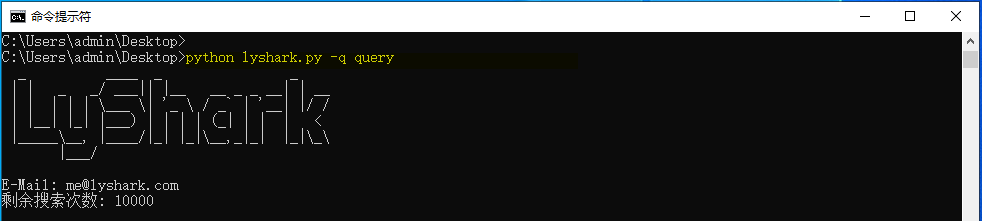# python 调用 R，使用rpy2

+关注继续查看

python 与 R 是当今数据分析的两大主流语言。作为一个统计系的学生，我最早接触的是R，后来才接触的python。python是通用编程语言，科学计算、数据分析是其重要的组成部分，但并非全部；而R则更偏重于统计分析，毕竟R是统计学家发明的，本身就是为统计而生。python的优势在于其全能性，几乎所有的领域都有python的身影，而R则在统计及其相关领域非常专业。二者各有优势。那么这么好的两个东西，能不能结合到一起呢？答案是肯定的。要想实现这种功能，一般必须要提供相应的调用接口。rpy2这个第三方库就提供了python调用R的接口。本文主要介绍rpy2的简单使用。

其实，在前一段时间，我就曾经尝试过安装rpy2，但是只在linux环境下安装成功了，在windows下总是安装失败。直到最近，我发现了一个非官方的python第三方库下载网址：

http://www.lfd.uci.edu/~gohlke/pythonlibs。这个网址提供的第三方库还是比较全的，而且是都是编译好的.whl文件，各个版本的都有，比如Pymediea，rpy2等比较难找的库这里都有，所以特此在这里分享给大家。找到rpy2这个库，由于我的python版本是2.7 64位，所以选择http://www.lfd.uci.edu/~gohlke/pythonlibs/tuoh5y4k/rpy2-2.7.8-cp27-none-win_amd64.whl这个地址下载。下载完成之后，解压，将解压后的全部文件放入python的site-packages目录下。还要做的一件重要的事情是增加环境变量R_HOME，将其值设置为电脑中R的安装路径。设置好了之后，发现 import rpy2 就可以正常导入了。

常用的命令：

1. import rpy2.robjects as robjects    这个命令是导入 r对象

2. robjects.r("r_script") 可以执行r代码，比如 pi = robjects.r('pi') 就可以得到 R 中的PI（圆周率），返回的变量pi是一个向量，或者理解为python中的列表，通过

pi 就可以取出圆周率的值。

3. robjects.r.source(“file.r”)可以执行r脚本文件。例子如下：

robjects.r.source('plot_demo.r')

plot_demo.r 内容如下：

# R 语言测试脚本
x <- c(1,2,3,4)
y <- x*x
jpeg(file="plot.jpg") # 保存图像
plt <- plot(x,y) # 画散点图
dev.off() # 关闭设备

a = robjects.r('a<-c(1,2,3)')
print(a)

x = robjects.r('x')
y = robjects.r('y')
print(x)
print(y)


运行得到：

 1 2 3 4

 1 4 9 16

robjects.FloatVector()等，看这些名字基本就知道干嘛的了。举例如下：

print(robjects.IntVector([1,2,3]))
print(robjects.FactorVector(['a','a','b','c']))
print(robjects.FloatVector([1.2,2.3]))
print(robjects.baseenv) # 基本环境空间
print(robjects.DataFrame({'a':[1,2],'b':[3,4]}))

 1 2 3

 a a b c

Levels: a b c

 1.2 2.3

<environment: namespace:base>

a.1L a.2L b.3L b.4L

1 1 2 3 4

r_script = '''
library(randomForest) # 导入随机森林包
## use data set iris
data = iris # 使用鸢尾花数据集
table(data$Species) ## create a randomForest model to classfy the iris species # 创建随机森林模型给鸢尾花分类 iris.rf <- randomForest(Species~., data = data, importance=T, proximity=T) print('--------here is the random model-------') print(iris.rf) print('--------here is the names of model-----') print(names(iris.rf)) confusion = iris.rf$confusion
print(confusion)
'''
robjects.r(r_script)

randomForest 4.6-12
Type rfNews() to see new features/changes/bug fixes.
 "--------here is the random model-------"

Call:
randomForest(formula = Species ~ ., data = data, importance = T, proximity = T)
Type of random forest: classification
Number of trees: 500
No. of variables tried at each split: 2

OOB estimate of error rate: 4%
Confusion matrix:
setosa versicolor virginica class.error
setosa 50 0 0 0.00
versicolor 0 47 3 0.06
virginica 0 3 47 0.06
 "--------here is the names of model-----"
 "call" "type" "predicted" "err.rate"
 "importance" "importanceSD" "localImportance" "proximity"
 "ntree" "mtry" "forest" "y"
 "test" "inbag" "terms"
setosa versicolor virginica class.error
setosa 50 0 0 0.00
versicolor 0 47 3 0.06
virginica 0 3 47 0.06|
8月前
|
Python
Ｃ调用Python崩溃的记录
Ｃ调用Python崩溃的记录
105 0
|
8月前
|

110 0
|
8月前
|
Python
C调用Python的示例代码
C调用Python的示例代码
57 0
|
8月前
|
Java Python
Python：jpype模块调用Java函数
Python：jpype模块调用Java函数
56 0
|
9月前
|
Python
python之面向对象中子类调用父类的构造方法
python之面向对象中子类调用父类的构造方法
93 0
|
9月前
|

Python 调用Zoomeye搜索接口

153 0